# 数据结构和算法

## 前言

### 1.数据结构的内容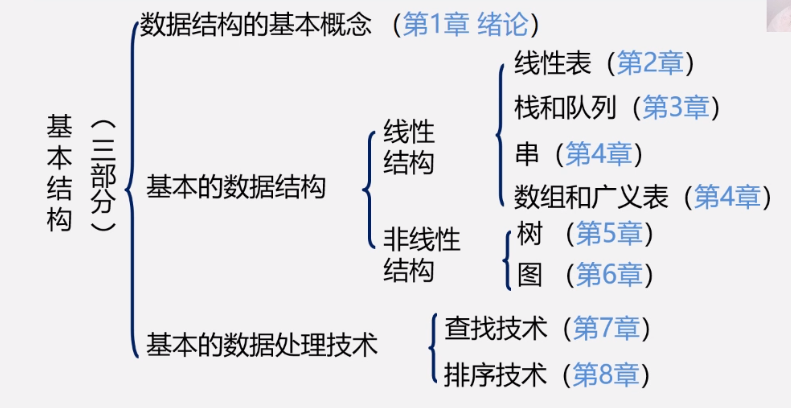### 2.数据结构的重要性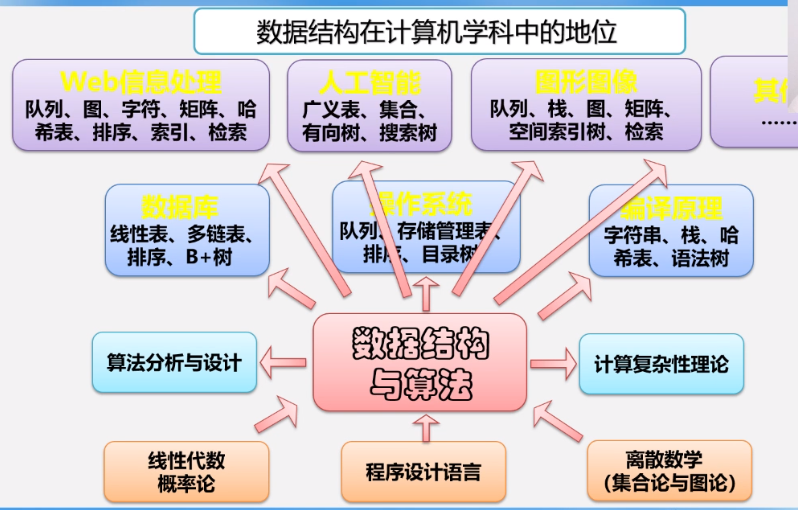• 数据结构是计算机软件相关专业的专业基础课
• 在教学计划中的地位：核心、承上启下的课程
• 数据结构是介于数学、计算机硬件和计算机软件三者之间的一门核心课程；
• 类似于武术中的==“练功”==科目。

## 一、绪论

### 1.1 数据结构的研究内容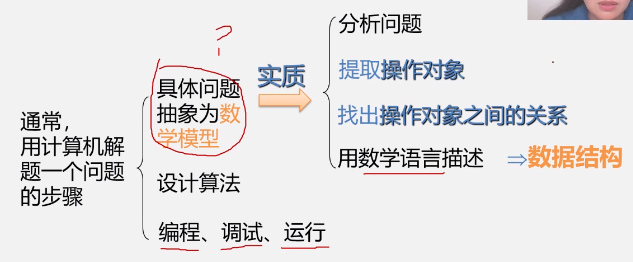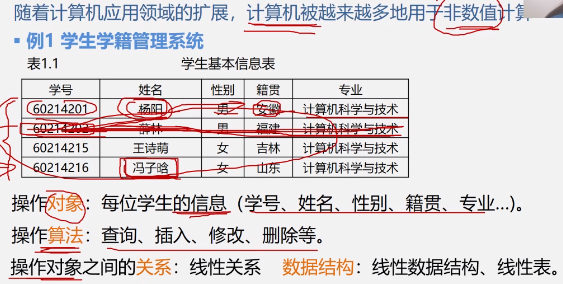==数据结构==是一门研究非数值计算的程序设计中计算机的操作对象以及它们之间的关系操作的学科。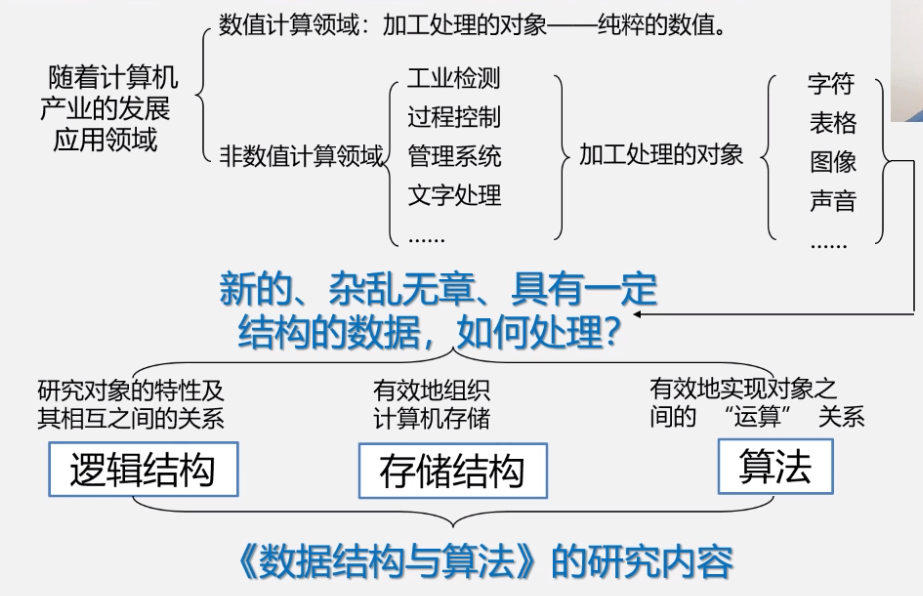### 1.2 基本概念和术语

#### 1.2.1 数据（Data）

• 能输入计算机并且能被计算机处理的各种符号的集合

• 是信息的载体
• 是对客观事物符号化的表示
• 能够被计算机识别、存储和加工
• 包括：

• 数值型的数据：整数、实数等
• 非数值型的数据：文字、图像、图形、声音

#### 1.2.2 数据元素（Data Element）和数据项（Data Item）

• 是数据的基本单位，在计算机程序中通常作为一个整体来进行考虑和处理。我们知道，数据当中其中有一部分关系比较紧密，我们通常作为整体来操作。如下表中一个学生的学号、姓名、性别、出生日期、政治面貌组成了一个数据元素。
• 也简称元素，或称为记录、结点或顶点。
• 一个数据元素（Data Element）可由若干个数据项（Data Item）组成。如下表中学号“0001”就是一个数据项。

0001张三1986/02/03团员
0002李四1978/11/28党员
00033王乐1992/08/12群众

#### 1.2.3 数据、数据元素、数据项之间的关系

• 数据 > 数据元素 > 数据项（例：学生表 > 个人记录 > 学号；）

#### 1.2.4 数据对象 （Data Object）

• 性质相同的数据元素的集合，是数据的一个子集。
• 数据元素与数据对象

• 数据元素——组成数据的基本单位：

• 与数据的关系：是集合的个体
• 数据对象——性质相同的数据元素的集合

• 与数据的关系：集合的子集

#### 1.2.5 数据结构（Data Structure）

##### 1.2.5.1 数据结构的概念
• 数据元素不是孤立存在的，它们之间存在着某种关系，数据元素相互之间的关系称为“结构”
• 是指相互之间存在一种或多种特定关系的数据元素的集合；
• 或者说，数据结构是带结构的数据元素的集合；
• 数据结构包括以下三个方面内容：

• 数据元素之间的逻辑关系，也称为逻辑结构
• 数据元素及其关系在计算机内存中的表示（又称为映像），成为数据的物理结构或数据的存储结构
• 数据的运算和实现，即对数据元素可以施加的操作以及这些操作在相应的存储结构上的实现。
##### 1.2.5.1 数据结构的两个层次
• 逻辑结构：

• 描述数据元素之间的逻辑关系
• 与数据的存储无关，独立于计算机
• 是从具体问题抽象出来的数学模型
• 物理结构（存储结构）：

• 数据元素及其关系在计算机存储器中的结构（存储方式）
• 是数据结构在计算机中的表示
• 逻辑结构与存储结构的关系

• 存储结构是逻辑关系的映像与元素本身的映像（既存储元素本身，又存储逻辑关系）
• 逻辑结构是数据结构的抽象，存储结构是数据结构的实现
##### 1.2.5.3 逻辑结构的种类
• 划分方法1：

• 线性结构：有且只有一个开始和一个终端结点，并且所有结点都最多只有一个直接前趋和一个直接后继。例如：线性表、栈、队列、串
• 非线性结构：一个结点可能有多个直接前趋和直接后继。例如：树、图
• 划分方法2：

• 集合：结构中的数据元素之间除了同属于一个集合的关系外，无其他任何关系；
• 线性结构：结构中的数据元素之间存在着一对一的关系；
• 树形结构：结构中的数据元素之间存在着一对多的层次关系；
• 图状结构或网状结构：结构中的数据元素之间存在着多对多的任意关系。
##### 1.2.5.4 存储结构的种类
• 顺序存储结构:用一组连续的存储单元依次存储数据元素，数据之间的逻辑关系由元素的存储位置来表示。C语言中使用数组来实现顺序存储结构。

!(https://cdn.kuetr.cn/2020/05/18/1589818568.png)

• 链式存储结构：用一组任意的存储单元存储数据元素，数据元素之间的逻辑关系用指针来表示。C语言中使用指针来实现链式存储结构。

!(https://cdn.kuetr.cn/2020/05/18/1589818582.png)

>   链式存储结构在存储数据的同时还存储了下一个元素的地址。

• 索引存储结构：在存储结点信息的同时，还建立附加的索引表。
• 散列存储结构：根据数据元素的关键字直接计算出该结点的存储地址。

#### 1.2.6 数据类型

##### 1.2.6.1数据类型的概念

==数据类型==是一组性质相同的值的集合以及定义域这个值集合上的一组操作的总称。

• 不仅有intcharfloatdouble等基本数据类型；
• 还有数组、结构体、共用体、枚举等构造数据类型
• 还有指针、空(void)类型
• 也可以使用typedef 自己定义、重命名现有的数据类型
• 高级语言中的数据类型明显地或隐含地规定了在程序执行期间变量的所有可能的取值范围，以及在这些数值范围上所允许进行的操作。
• 约束变量或常量的取值范围
• 约束变量或常量的操作

##### 1.2.7.1 抽象数据类型的概念

• 由用户定义，从问题抽象出数据模型（逻辑结构）
• 还包括定义在数据模型上的一组抽象运算（相关操作）
• 不考虑计算机内的具体存储结构与运算的具体实现算法
##### 1.2.7.2 抽象数据类型的形式定义

（此处需要离散数学的部分知识）

• 其中：

• D是数据对象;
• S是D上的关系集；
• P是对D的基本操作集。

ADT 抽象数据类型名{
数据对象:<数据对象的定义>
数据关系:<数据关系的定义>
基本操作:<基本操作的定义>
}
ADT 抽象数据类型名{
Data
数据对象的定义
数据元素之间逻辑关系的定义
Operation
操作1
初始条件
操作结果描述
操作2
……
操作n
……
}ADT 抽象数据类型名

• 数据对象、数据关系的定义用伪代码描述。
• 基本操作的定义格式为：

基本操作名(参数表)
初始条件:<初始条件描述>
操作结果:<操作结果描述>

• 参数表：

• 赋值参数：只为操作提供输入值。
• 引用参数以&打头，除可提供输入值外，还将返回操作结果。
• 初始条件：描述操作执行之前数据结构和参数应满足的条件，若不满足，则操作失败，并返回相应出错信息。若初始条件为空，则省略。
• 操作结果：说明操作正常完成之后，数据结构的变化状况和应返回的结果。

ADT Circle{
数据对象:D={r,x,y|r,x,y均为实数}
数据关系:E={<r,x,y>|r是半径,<x,y>是圆心坐标}
Circle(&C,r,x,y)  //构造一个圆
操作结果：构造一个圆
double Area(C)  //求圆的面积
初始条件：圆已存在
操作结果：计算面积
double Circumference(C)//求圆的周长
初始条件：圆已存在
操作结果：计算周长
}ADT Circle

ADT Complex{
D = {r1,r2|r1,r2都是实数}
S = {<r1,r2>|r1是实部,r2是虚部}
assign(&C,v1,v2)
初始条件:空的负数已存在
操作结构:构造复数C，r1,r2分别被赋以参数v1,v2的值。
destory(&C)
初始条件：复数C已存在
操作结果：复数C被销毁。
……
}ADT Complex

#### 1.2.8 总结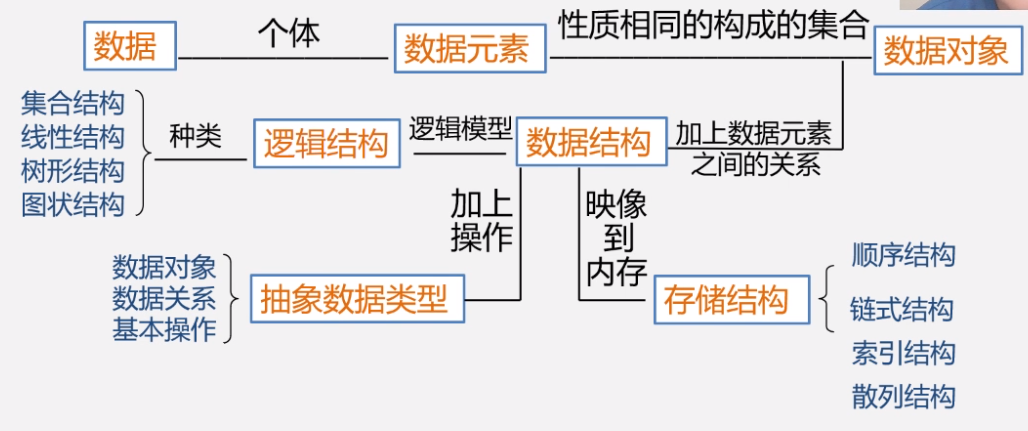### 1.3抽象数据类型的表示与实现

#### 1.3.1 抽象数据类型如何实现

• 即利用处理器已存在的数据类型来说明新的结构，用已经实现的操作来组合新的操作。

### 1.4 算法和算法分析#### 1.4.1 算法的定义

• 对特定问题求解方法和步骤的一种描述，它是指令的有限序列。其中每个指令表示一个或多个操作。简而言之，算法就是解决问腿的方法和步骤。

#### 1.4.2 算法的描述方法

• 自然语言
• 流程图：传统流程图、NS流程图
• 伪代码：类C语言
• 程序代码

#### 1.4.3 算法与程序

• 算法是解决问题的一种方法或一个过程，考虑如何将输入转换成输出，一个问题可以有多种算法。
• 程序是用某种程序设计语言对算法的具体实现；

• 程序 = 数据结构 + 算法；
• 数据结构通过算法实现操作，算法根据数据结构设计程序。

#### 1.4.4 算法特征

• 有穷性：一个算法必须总是在执行有穷步之后结束，且每一步都在有穷时间内完成；
• 确定性：算法中的每一条指令必须有确切的含义，没有二义性，在任何条件下，只有唯一的一条执行路径，即对于相同的输入只能得到相同的输出；
• 可行性：算法是可执行的，算法描述的操作可以通过已经实现的基本操作执行有限次来实现；
• 输入：一个算法有零个或多个输入；
• 输出：一个算法有一个或多个输出。

#### 1.4.5 算法设计的要求

• 正确性（Correctness）

• 程序中不含语法错误
• 程序对于几组输入数据能够得出满足要求的结果；
• 程序对于精心选择的、典型、苛刻且带有刁难性的几组输入数据能够得出满足要求的结果；（√）
• 程序对于一切合法的输入数据都能得出满足要求的结果。
• 通常以第三层意义上的正确性作为衡量一个算法是否合格的标准

• 算法主要是为了人的阅读和交流，其次才是计算机执行，因此算法应该易于人的理解
• 另一方面，晦涩难读的算法易于隐藏较多错误而难以调试
• 健壮性（Robustness）

• 当输入非法数据时，算法恰到的作出反应或进行相应处理，而不是产生莫名其妙的输出结果
• 处理出错的方法，不应是中断程序的执行，而应是返回一个表示错误或错误性质的值，以便在更高的抽象层次上进行处理。（异常处理）
• 高效性（Effeciency）

• 要求花费尽量少的时间和尽量低的存储需求

#### 1.4.6 算法分析

• 时间效率：指的是算法所耗费的时间
• 空间效率：指的是算法执行过程中所耗费的存储空间

##### 1.4.6.1 算法时间效率

###### （1）算法效率的两种度量方法
• 事后统计

将算法实现，测算其时间和空间开销。

缺点：（1）编写程序实现算法将花费较多的时间和精力；（2）所得实验结果依赖于计算机的软硬件等环境因素，掩盖算法本身的优劣。

• 事前分析

对算法所消耗资源的一种估算方法。

###### （2）事前分析法简述

• 大致可以等于计算机执行一种简单的操作（如赋值、比较、移动等）所需的时间与算法中进行的简单操作次数乘积
• 也即算法中每条语句的执行时间之和

**算法运行时间 = $\sum$每条语句的执行次数 $\times$ 该语句执行一次所需的时间**

每条语句的执行次数也叫做语句频度，因此：

**算法运行时间 = $\sum$每条语句频度 $\times$ 该语句执行一次所需的时间**

>   每条语句执行一次所需的时间，一般是随及其而异的。取决于机器的指令性能、速度以及编译的代码质量。是由机器本身软硬件环境决定的，与算法无关。
>
>   所以，我们可以假设执行每条语句所需的时间均为单位时间。此时对算法的运行时间的讨论就可转化为讨论该算法中所有语句的执行次数，即频度之和了。

即：

**算法运行时间 $\approx \sum$每条语句频度**

从而脱离及其的软硬件环境来分析算法的时间性能。


==我们把算法所耗费的时间定义为该算法中每条语句的频度之和。==

###### （3）算法时间复杂度的渐进表示法
• 算法时间复杂度

• 最坏时间复杂度：指在最坏情况下，算法的时间复杂度
• 平均时间复杂度：指在所有可能输入实例在等概率出现的情况下，算法的期望运行时间
• 最好时间复杂度：指在最好情况下，算法的时间复杂度
• ==一般总是考虑在最坏情况下的时间复杂度，以保证算法的运行时间不会比它更长。==
• 对于复杂的算法，可以将它分成几个容易估算的部分，然后利用大O运算规则，计算算法的时间复杂度。

• 加法规则：$T(n)=T1(n)+T2(n)=o(f(n))+o(g(n))=o(max(f(n),g(n)))$
• 乘法规则：$T(n)=T1(n)\times T2(n)=o(f(n))\times o(g(n))=o(f(n)\times g(n))$。
• 为了便于比较不同算法的时间效率，我们仅比较它们的数量级。
• 若有某个辅助函数$f(n)$，使得当$n$趋近于无穷大时，$\frac{T(n)}{f(n)}$的极限值为不等于零的常数，则称$f(n)$是$T(n)$的同数量级函数。记作$T(n)=o(f(n))$，称$o(f(n))$为算法的渐进时间复杂度，简称时间复杂度
• ==一般情况下，不必计算所有操作的执行次数，而只考虑算法中基本操作执行的次数==，它是问题规模n的某个函数，用$T(n)$表示。
• 算法中基本语句重复执行的次数问题规模$n$的某个函数$f(n)$，算法的时间量度记作：$T(n)=o(f(n))$。

• 它表示随着n增大，算法执行的时间增长率和$f(n)$的增长率相同，称渐进时间复杂度
• 基本语句是指算法中重复执行次数和算法的执行时间称正比的语句，它对算法运行时间的贡献最大，执行次数最多。
• 问题规模$n$越大，执行时间越长。

• 排序：$n$为记录数
• 矩阵：$n$为矩阵的阶数
• 多项式：$n$为多项式的项数
• 集合：$n$为元素个数
• 树：$n$为树的结点个数
• 图：$n$为图的定点数或边数

###### （5）分析算法时间复杂度的基本方法
• 找出语句频度最大的那条语句作为基本语句
• 计算基本语句的频度得到问题规模$n$的某个函数$f(n)$
• 取其数量级用符号O表示。
##### 1.4.6.2 算法时间效率的比较
• 当$n$取得很大时，指数时间算法和多项式时间算法在所需时间上非常悬殊。
• 时间复杂度$T(n)$按数量级递增顺序为：

$o(1)$$o(\log _2 n)$$o(n)$$o(nlog_2n)$$o(n^2)$$o(n^3) o(n^k)$$o(2^n)$

#### 1.4.7 渐进空间复杂度

• 空间复杂度：算法所需存储空间的度量，记作:$S(n)=O(f(n))$，其中$n$为问题的规模（或大小）。
• 算法要占据的空间：

• 算法本身要占据的空间，输入/输出、指令、常数、变量等。
• 算法要使用的辅助空间。

#### 1.4.8 设计好算法的过程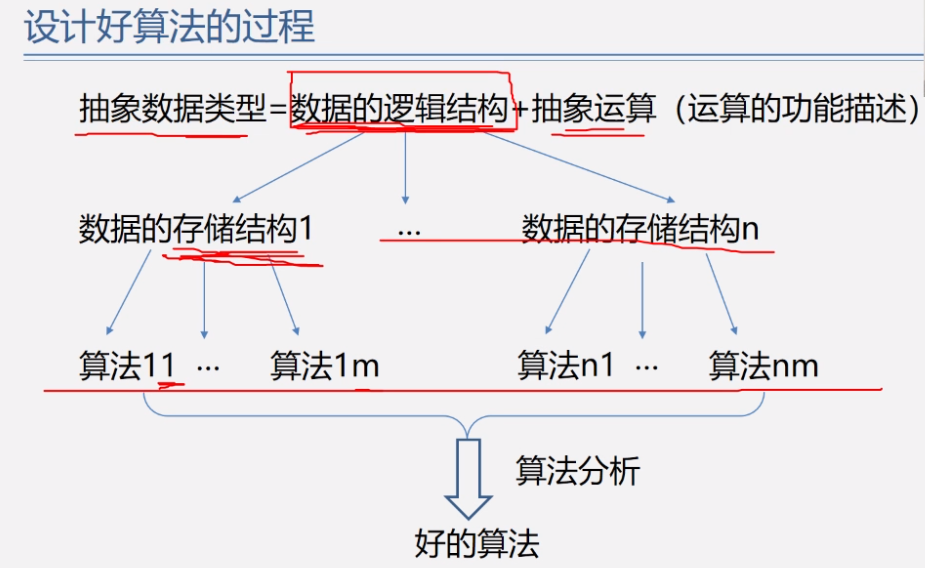Last modification：May 19th, 2020 at 12:22 am